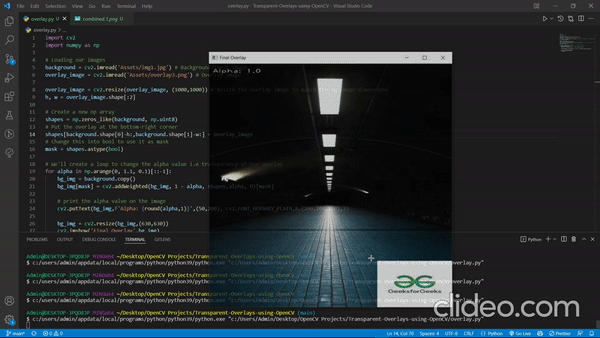Open in App
Not now

# Transparent overlays with Python OpenCV

• Last Updated : 29 Mar, 2022

In this article, we are going to see how to Transparent overlays with Python OpenCV.

For this program to work, first we’ll need two inputs: Background Image, Overlay Image. We’ll then create a NumPy array with the same dimension as the background image, with all values as 0. Then we’ll create a mask using this array and the overlay.

Now the main part of our program the overlay will be done by using the cv2.addWeighted() method.

cv2.addWeighted() :This method basically calculates the average weighted sum of the two input arrays to return an output array.

## Python3

 `import` `cv2` `import` `numpy as np`   `# Loading our images` `# Background/Input image` `background ``=` `cv2.imread(``'Assets/img1.jpg'``)  `   `# Overlay image` `overlay_image ``=` `cv2.imread(``'Assets/overlay3.png'``)  `   `# Resize the overlay image to match the bg image dimensions` `overlay_image ``=` `cv2.resize(overlay_image, (``1000``, ``1000``))` `h, w ``=` `overlay_image.shape[:``2``]`   `# Create a new np array` `shapes ``=` `np.zeros_like(background, np.uint8)`   `# Put the overlay at the bottom-right corner` `shapes[background.shape[``0``]``-``h:, background.shape[``1``]``-``w:] ``=` `overlay_image`   `# Change this into bool to use it as mask` `mask ``=` `shapes.astype(``bool``)`   `# We'll create a loop to change the alpha` `# value i.e transparency of the overlay` `for` `alpha ``in` `np.arange(``0``, ``1.1``, ``0.1``)[::``-``1``]:` `  `  `    ``# Create a copy of the image to work with` `    ``bg_img ``=` `background.copy()` `    ``# Create the overlay` `    ``bg_img[mask] ``=` `cv2.addWeighted(bg_img, ``1` `-` `alpha, shapes,` `                                   ``alpha, ``0``)[mask]`   `    ``# print the alpha value on the image` `    ``cv2.putText(bg_img, f``'Alpha: {round(alpha,1)}'``, (``50``, ``200``),` `                ``cv2.FONT_HERSHEY_PLAIN, ``8``, (``200``, ``200``, ``200``), ``7``)`   `    ``# resize the image before displaying` `    ``bg_img ``=` `cv2.resize(bg_img, (``630``, ``630``))` `    ``cv2.imshow(``'Final Overlay'``, bg_img)` `    ``cv2.waitKey(``0``)`

Output:My Personal Notes arrow_drop_up
Related Articles